# Low Interest Rates And High Valuations

Lower interest rates justify a higher than average price-earnings valuation." - Pundit

You've heard the story before:

Equity valuations are high…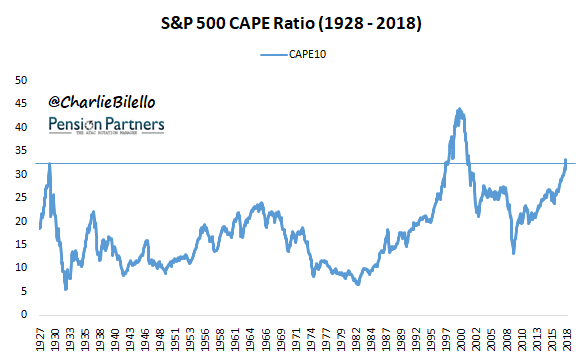Interest rates are low…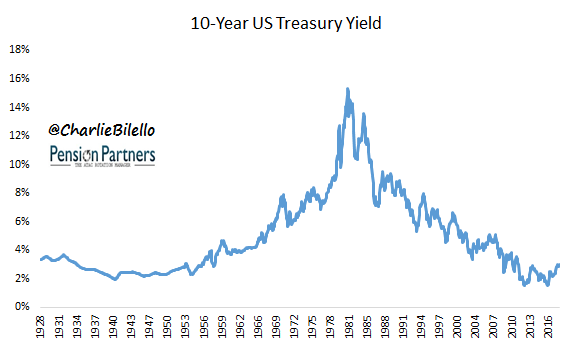Data sources for all charts/tables herein: Robert Shiller

Ergo, high valuations are "justified" by lower interest rates. Why?

Some argue that because stocks are the present value of future cash flows, lower interest rates should result in a higher present value, and vice versa (PV = FV / (1+i)^n, where i = interest rates).

Others argue that when bond yields are lower, earnings yields (Earnings/Price) should be lower as well. Investors are said to be choosing between stocks and bonds (they are "competing" asset classes), and if they are accepting a lower interest rate on bonds, they should also accept a lower yield on stocks. The corollary: they will accept a higher valuation (Price/Earnings).

Putting aside whether these arguments hold water, what does the data suggest? Is there a strong relationship between interest rates and valuations? Let's take a look…

Going back to 1881, there is little evidence of a strong relationship. The R^2 between the two variables (CAPE Ratio and 10-Year Treasury Yield) is .025, which means that knowledge of interest rates accounts for only 2.50% of the variation in CAPE ratios. This would be essentially useless for predictive purposes, as 97.50% of the variation in CAPE ratios occurs for reasons unconnected to interest rates.

Breaking 10-year yields down into deciles, one can readily observe the lack of a linear relationship…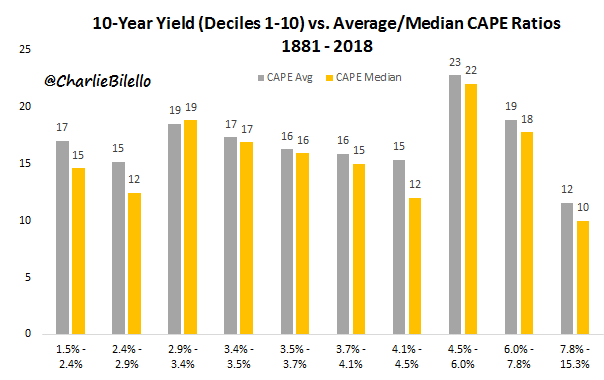The lowest decile of interest rates (1.5-2.4%) actually has a median CAPE Ratio of 15, which is slightly below the overall median of 16.

The highest average CAPE ratios historically were found not in periods of extremely low interest rates, but in the 8th decile (range of 4.5% to 6% 10-Year Treasury yield).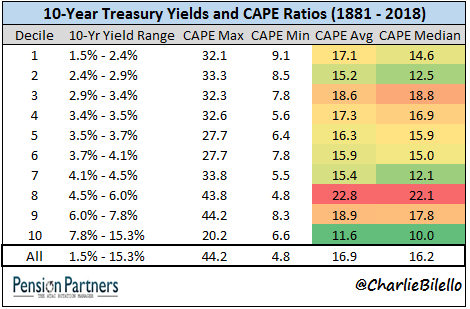But what about the story of the early 1980s, the one where interest rates started at record highs and were accompanied by extremely low valuations? Yes, this is a good one, and over the next 18 years, stock prices and valuations would rise while interest rates would fall.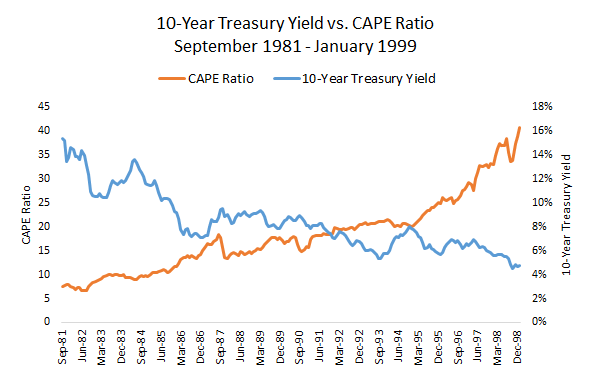The only problem is this story is rarely followed by the period from 1949 to 1968 when valuations and interest rates moved higher, together.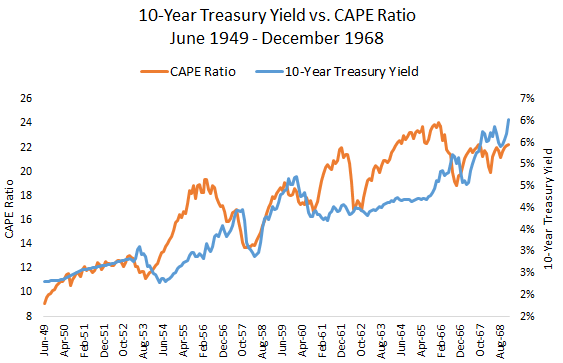The full data set shows a correlation of -0.16, meaning that there is a slight historical tendency for higher interest rates to be associated with lower valuations (and vice verse), but it is not nearly as predictive as many suggest. There have been many periods where interest rates and valuations have been low (1934-35, 1938, 1940-1954) and other periods where interest rates and valuations have been high (1995-2000). There is no calculation that can give you the appropriate valuation at a given interest rate because such a valuation does not exist.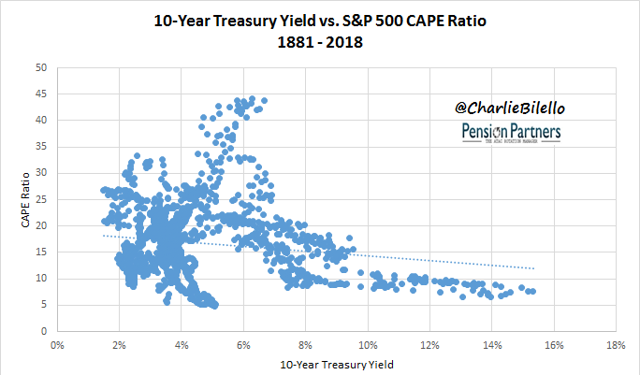Why then, do market pundits continue to perpetuate the myth that interest rates are the primary driver of equity valuations? Likely because it helps them with a narrative. If they are bullish on the stock market and want to dismiss higher valuations as irrelevant, low interest rates seem to be the perfect rationale.

Few will confront them with the historical evidence, and fewer still will ask the most important question of all: if high valuations are "justified" due to low interest rates, does that imply that forward returns are expected to be strong? I'll explore the answer to that question in an upcoming post.